# OR Gate

The OR gate is a simple logic gate but it can be built in several different ways. In its simplest form, it requires only two transistors but when using it to send a signal it will require three or more transistors. It is also called an inclusive OR gate since the output is on when both inputs are on. Most of the time it is just called an OR gate though. The exclusive OR gate is only on when one of the inputs is on.

## OR Gate 1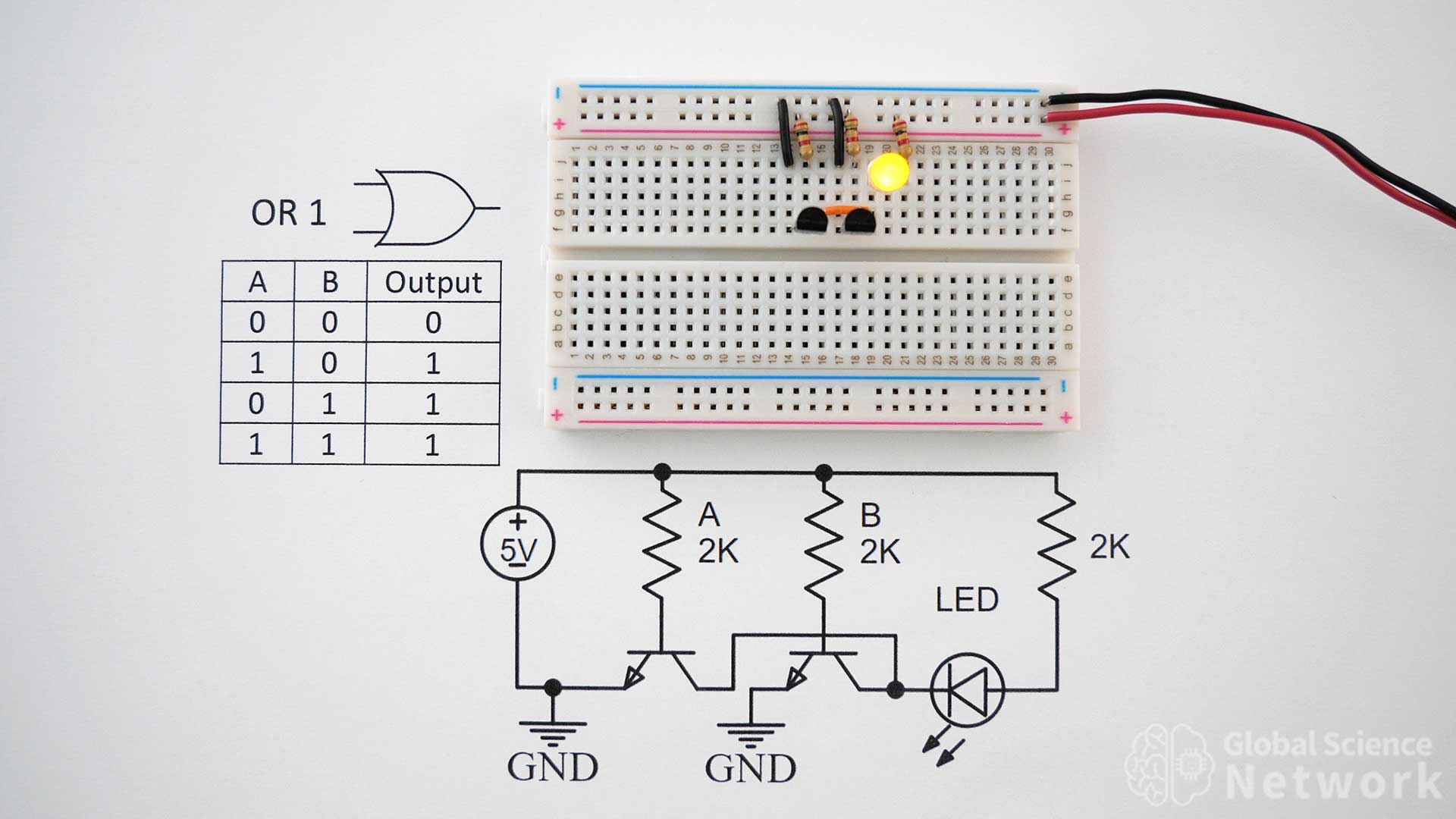The circuit above shows the simplest OR Gate. It only requires two transistors, three resistors, two ground wires, a jumper wire, and a LED. In the photo, both inputs are on which is why the output is on. The only case where the output will be off is when both inputs are off. An input is turned off by removing resistor A or resistor B.

The video above shows how to build all types of logic gates. At 22:44 the OR gate is discussed in detail. It does show the four different ways of building an OR gate including using NAND and NOR gates.

### OR Gate Truth Table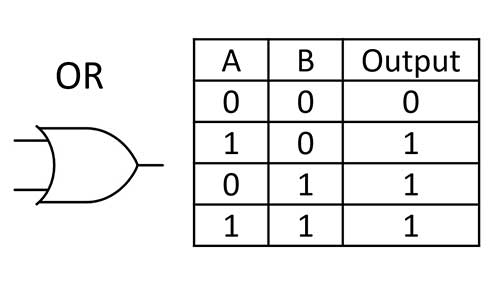The symbol and truth table for an OR gate is shown above. Looking at the truth table it is clear that when both inputs are off the output is off. In all other cases, the output will be on. How an OR gate is made does not change the truth table. All four OR gate circuit configurations will have the same truth table.

### OR Gate 1 Circuit DiagramThe circuit diagram for the simplest case is shown above. Input A and input B are connected using 2K resistors. Note the direction of the LED is such that the current is flowing into the circuit rather than as an output. This means the circuit only works by itself or as the last OR gate in a larger circuit.

## OR Gate 2OR gate 2 is very similar to OR gate 1 but an additional transistor is added on the right-hand side of the circuit. This transistor is set up similarly to an inverter however the director of the LED is also flipped. Since the current was sent out rather than through the circuit and the signal was inverted the logic of the output remains the same. Looking at the circuit both inputs A and B are on which is why the output is on. The output LED will only be off when neither input resistor is connected.

## OR Gate 2 Circuit Diagram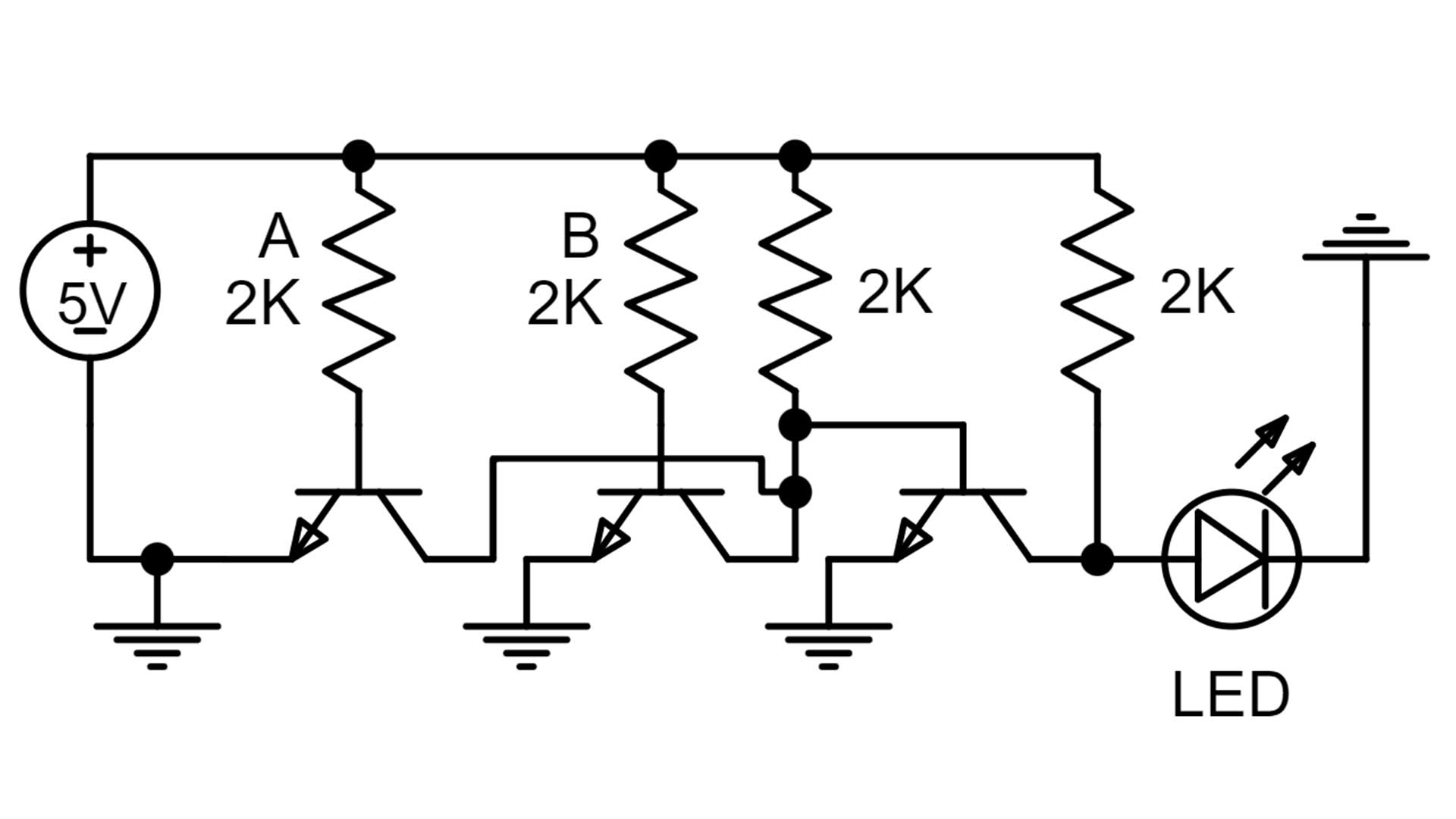The circuit diagram for AND gate 2 is shown above. This shows the connections of each individual component. The circuit requires three transistors, four 2K resistors, connecting wires, and an LED. Resistor values from 330 ohms to 2K will work. The direction of the LED is going out of the circuit. This is a good thing as the output can be sent to other logic gates or circuits.

## OR Gate Built with NAND Gates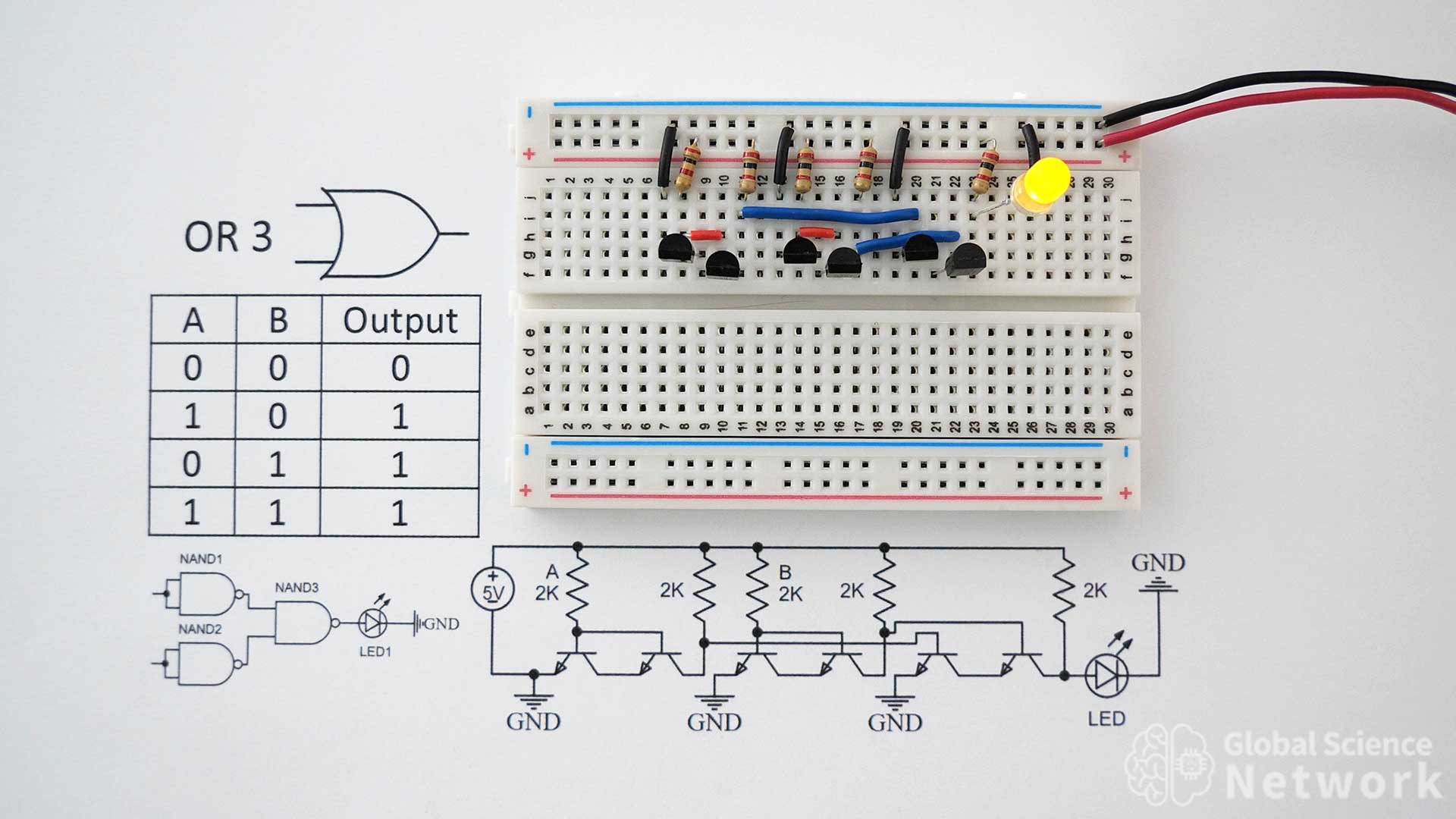OR gate 3 is built using three NAND gates. Each NAND gate requires two transistors which means that 6 transistors are used in total. Input A feeds into the base of both transistors in the first NAND gate. Input B feeds into the base of both transistors in the second NAND gate. In the photo, both inputs A and B are on which is why the input is on. The first resistor for the left is input A and the third resistor is input B. When both input resistors are removed it makes the inputs off and the output will also be off. As long as one input is on the output will be on.

### OR Gate Built with NAND Gates Circuit Diagrams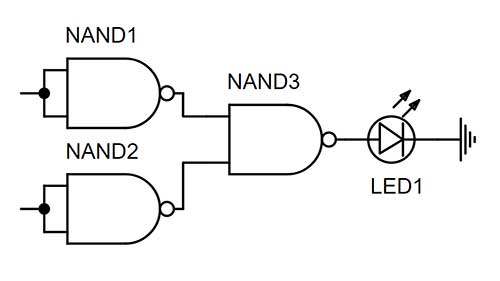The digital logic gate level circuit diagram for an OR gate built using NAND gates is shown above. This shows how the connections between the gates need to be made. It is a high-level diagram and does not include specifics such as resistor placement and ground connection placement for the NAND gates. Often times it is clear how the circuit works though by just looking at the logic gate level diagram.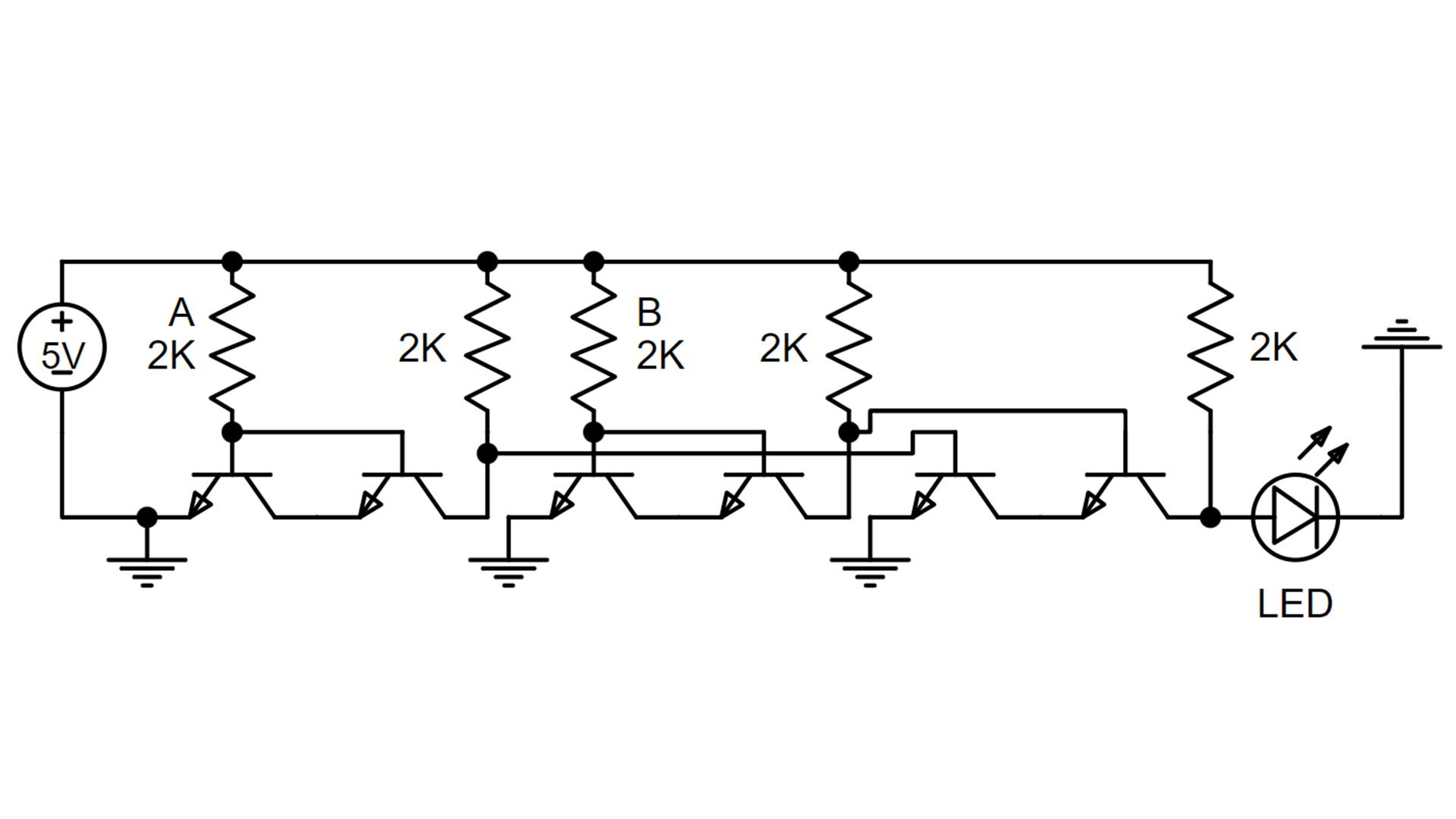Or gate 3 which was built using three NAND gates is shown in the circuit diagram above. All the connections for the six transistors can be seen clearly. The output is being sent outward from the circuit which means this logic gate design will work anywhere within a circuit design. It is more complicated than the three-transistor design so it will mostly be used when the computer logic is based on NAND gates only.

## OR Gate Built with NOR GatesOR gate 4 is built using two NOR gates. This circuit requires four transistors, four resistors, connection wires, and an LED. In the photo input A and input B are both connected which is why the output is ON. The output will only be off if both input connections are removed. Orange jumpers connect the collectors of two transistors in each NOR gate. Blue wires are used to connect the output of the first NOR gate to the inputs of the second NOR gate. The collector of every transistor is connected to the ground by using a black jumper wire.

### OR Gate Built with NOR Gates Circuit Diagrams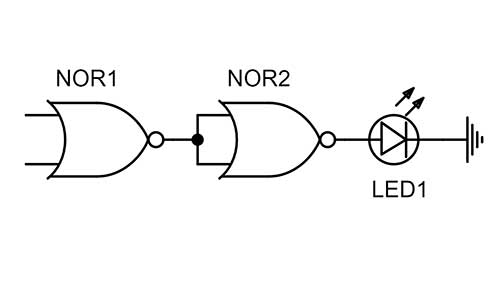The logic gate level circuit diagram of the OR gate built using NOR gates is shown above. This is a simple diagram that shows where the input and output connections should be made.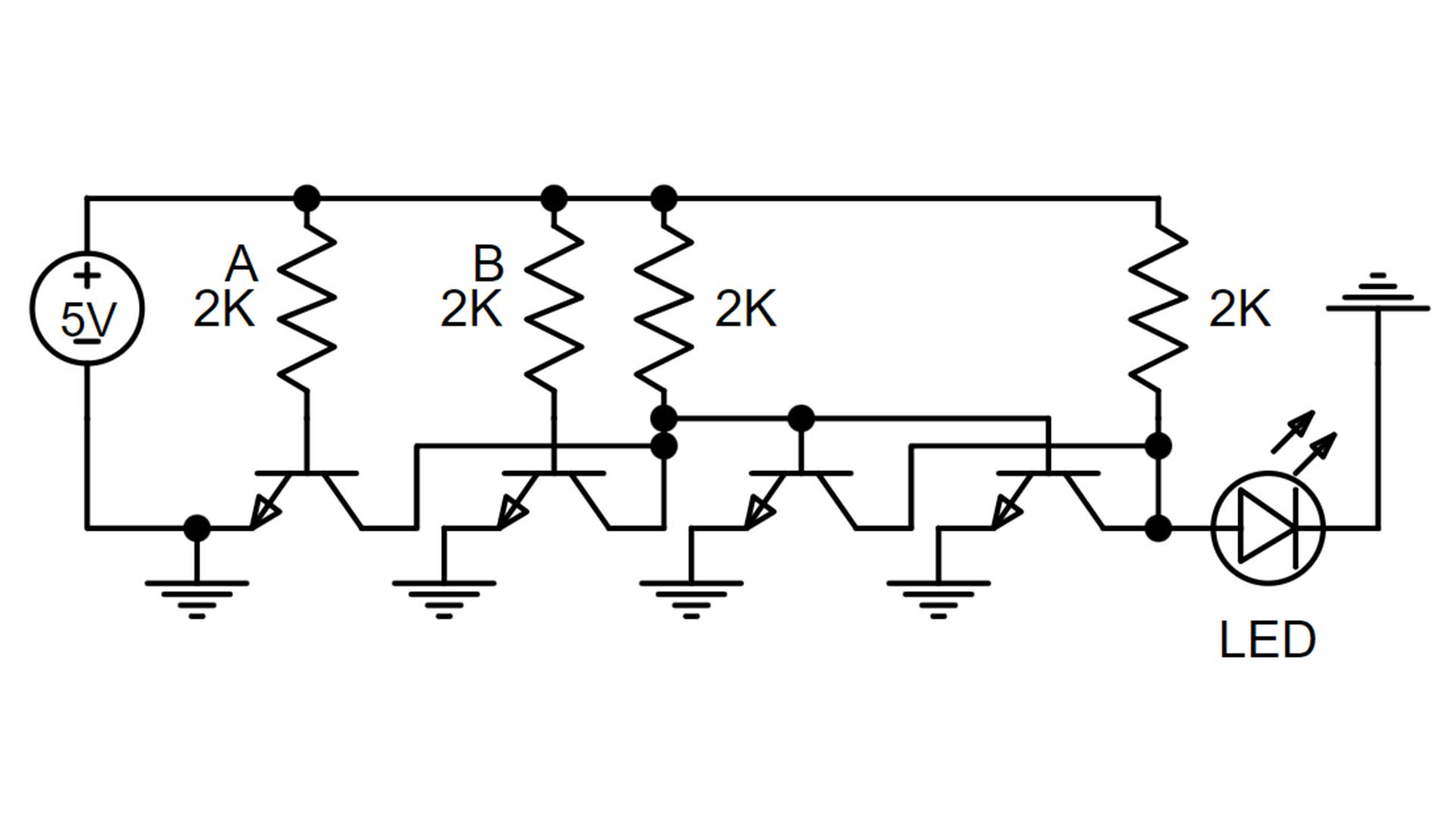The component level circuit diagram for OR gate 4 is shown above. This calls out that 2K resistors should be used as well as a 5-volt power supply. When I built the circuit I used a 5-volt battery pack that is typically used for charging cell phones. Any color LED could be used. Changing the resistor value will change the current resulting in a more bright or less bright LED. Using 330-ohm resistors will result in a bright LED and using 2.2K resistors will make a moderately bright LED.